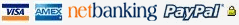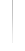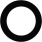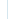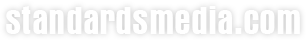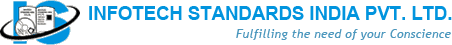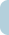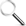Books Standards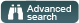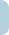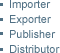Welcome Guest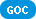|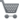0 items in your shopping cart

## BROWSE BY SUBJECTSAuditingEnergyEnvironment EngineeringPollutionMechanical EngineeringSafetyElectrical EngineeringEngineeringProject ManagementManagementMetallurgyCivil EngineeringChemicalManufacturingTQMPowerPetroleumSix SigmaLeanWeldingDrilling5STPMDie CastingUDCPressure Vessel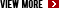## BROWSE BY PUBLISHERS***

## BROWSE BY STANDARDSAIAGAPIASMEASTMAWWABISBSICTIDINIECISONFPASAE

## BROWSE BY CATEGORY***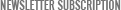Join our mailing list to recieve newsletters

# Digital Signal Processing Algorithms:Number Theory Convolution Fast Fourier Transforms & Application

Send to friend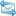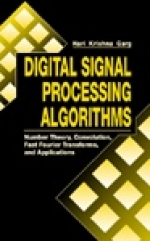Title: Digital Signal Processing Algorithms:Number Theory Convolution Fast Fourier Transforms & Application Author: Hari Krishna ISBN: 0849371783 / 9780849371783Format: Hard CoverPages: 1998Publisher: CRC PressYear: 1998Availability: 45-60 days Description Feature Contents Digital Signal Processing Algorithms describes computational number theory and its applications to deriving fast algorithms for digital signal processing. It demonstrates the importance of computational number theory in the design of digital signal processing algorithms and clearly describes the nature and structure of the algorithms themselves. The book has two primary focuses: first, it establishes the properties of discrete-time sequence indices and their corresponding fast algorithms; and second, it investigates the properties of the discrete-time sequences and the corresponding fast algorithms for processing these sequences.Digital Signal Processing Algorithms examines three of the most common computational tasks that occur in digital signal processing; namely, cyclic convolution, acyclic convolution, and discrete Fourier transformation. The application of number theory to deriving fast and efficient algorithms for these three and related computationally intensive tasks is clearly discussed and illustrated with examples. Its comprehensive coverage of digital signal processing, computer arithmetic, and coding theory makes Digital Signal Processing Algorithms an excellent reference for practicing engineers. The authors' intent to demystify the abstract nature of number theory and the related algebra is evident throughout the text, providing clear and precise coverage of the quickly evolving field of digital signal processing. • Covers all aspects of computational number theory and the related digital signal processing algorithms • Emphasizes the computational aspects of algorithms and discusses the theoretical analysis and mathematical properties of the algorithms • Describes the development of fast algorithms for cyclic convolution, acyclic convolution, and discrete Fourier transformation • Includes figures, flowcharts, and tables that emphasize concepts presented in the text • Describes the applications of digital signal processing algorithms to computer arithmetic and coding theory Acknowledgments Chapter 1 : Introduction Part 1 : Computational Number Theory Thoughts on Part I Chapter 2 : Computational Number Theory Chapter 3 : Polynomial Algebra Chapter 4 : Theoretical Aspects of the Discrete Fourier Transform and Convolution Chapter 5 : Cyclotomic Polynomial Factorization and Associated Fields Chapter 6 : Cyclotomic Polynomial Factorization in Finite Fields Chapter 7 : Finite Integer Rings : Polynomial Algebra and Cyclotomic Factorization Part 2 : Convolution Algorithms Thoughts on Part II Chapter 8 : Fast Algorithms for Acyclic Convolution Chapter 9 : Fast One-Dimensional Cyclic Convolution Algorithms Chapter 10 : Two-and Higher-Dimensional Cyclic Convolution Algorithms Chapter 11 : Validity of Fast Algorithms Over Different Number Systems Chapter 12 : Fault Tolerance for Integer Sequences Part 3 : Fast Fourier Transform (FFT) Algorithms Thoughts on Part III Chapter 13 : Fast Fourier Transform : One-Dimensional Data Sequences Chapter 14 : Fast Fourier Transform : Multidimensional Data Sequences Part 4 : Recent Results on Algorithms in Finite Integer Rings Thoughts on Part IV Paper 1 : A Number Theoretic Approach to Fast Algorithms for Two-Dimensional Digital Signal Processing in Finite Integer Rings Paper 2 : On Fast Algorithms for One-Dimensional Digital Signal Processing in Finite Integer and Complex Integer Rings Paper 3 : Cyclotomic Polynomial Factorization in Finite Integer Rings with Applications to Digital Signal Processing Paper 4 : Error Control Techniques for Data Sequences Defined in Finite Integer Rings Appendix A : Small Length Acyclic Convolution Algorithms Appendix B : Classification of Cyclotomic Polynomials IndexAbout Us | Contact us